Anúncio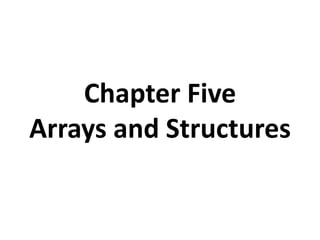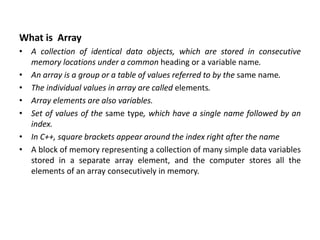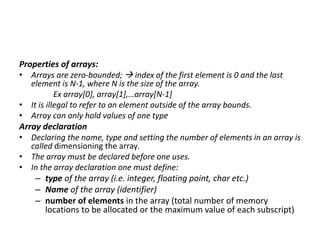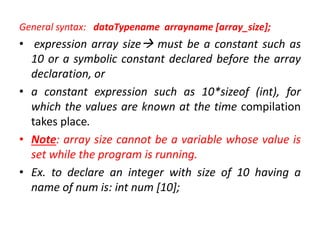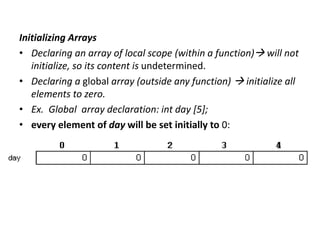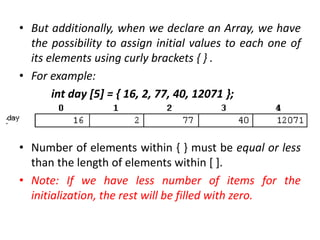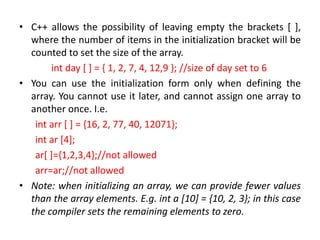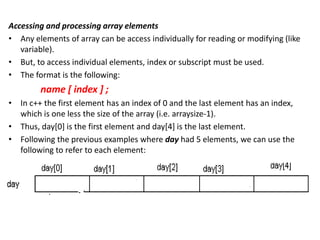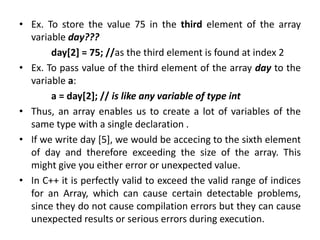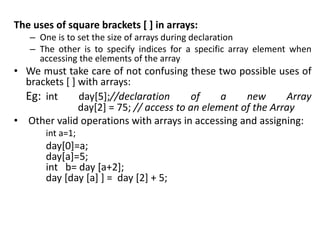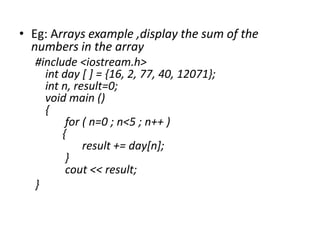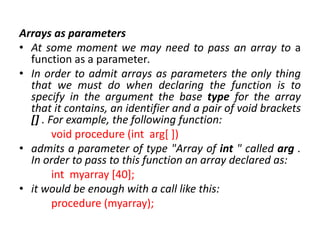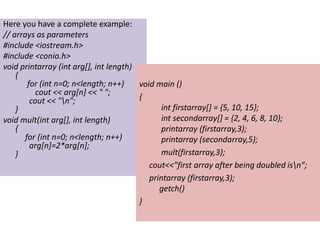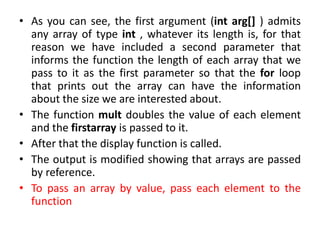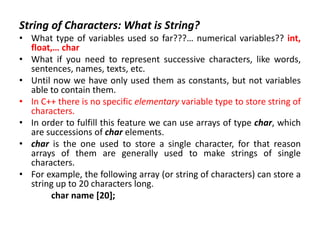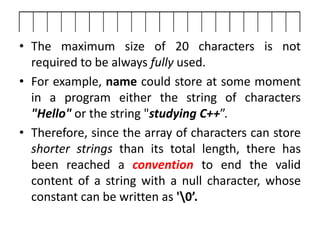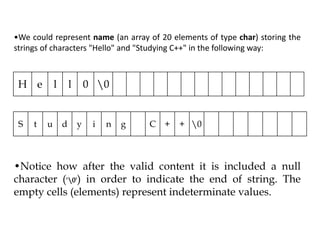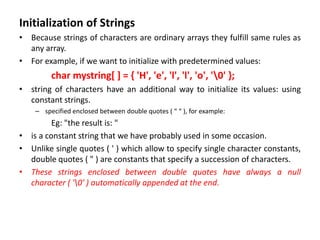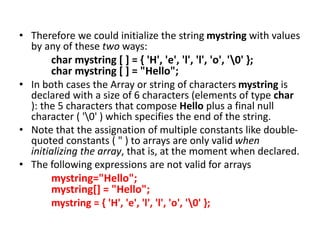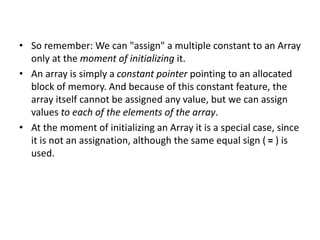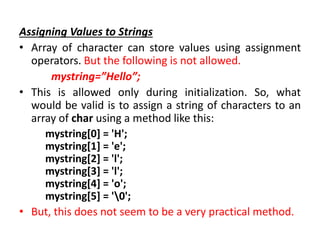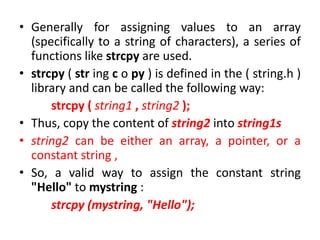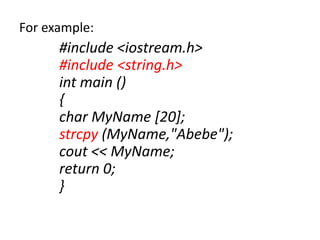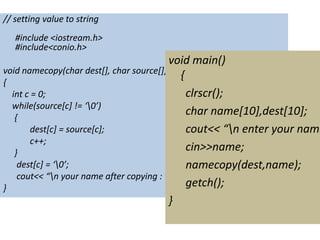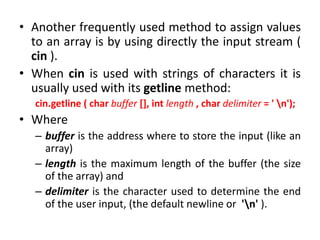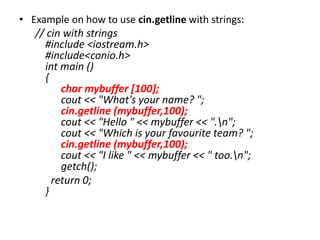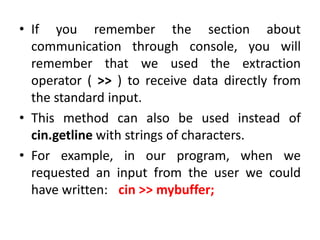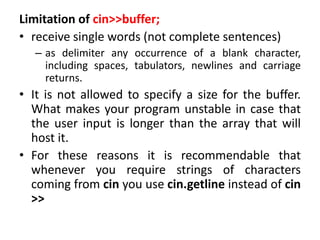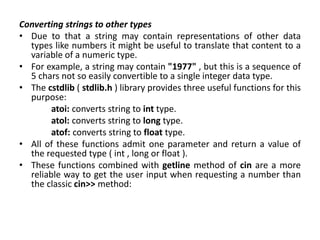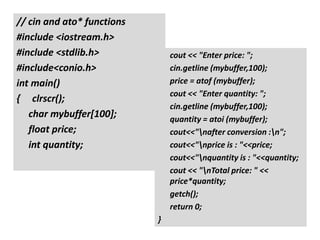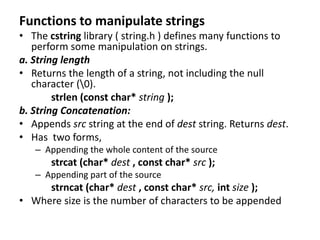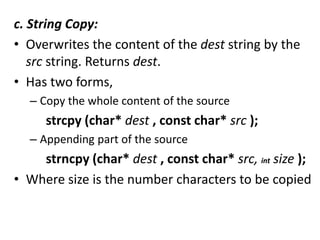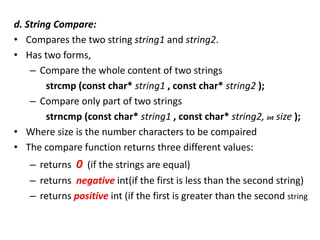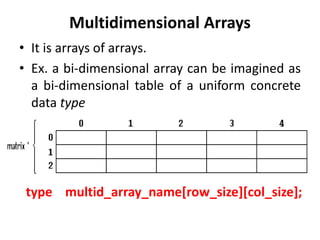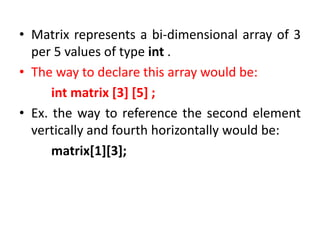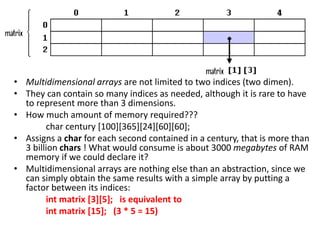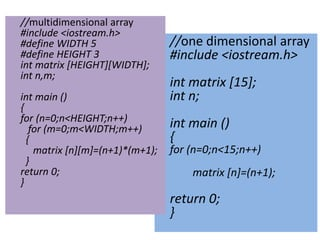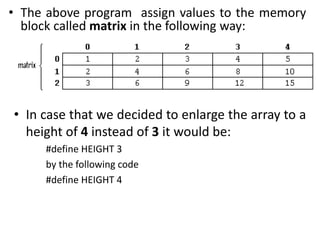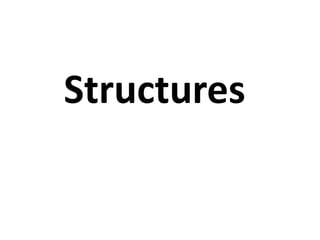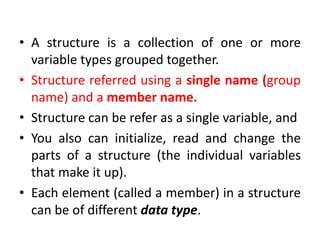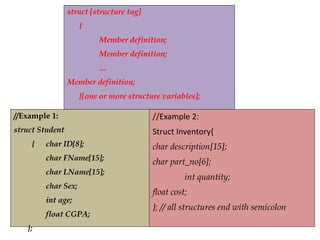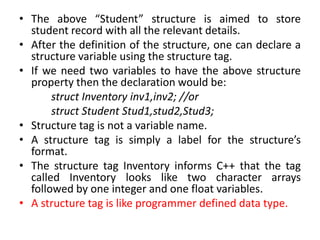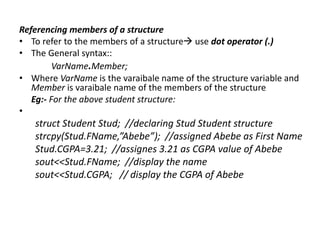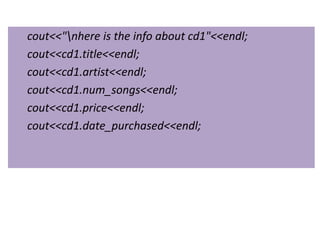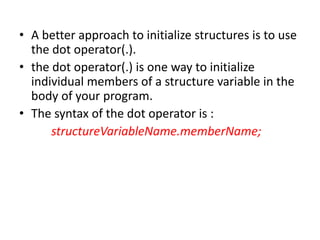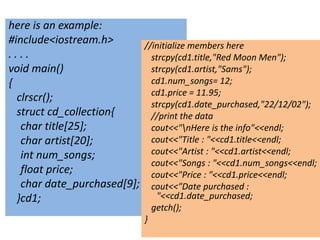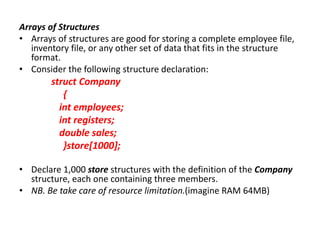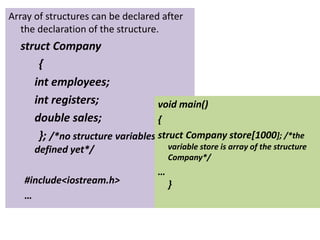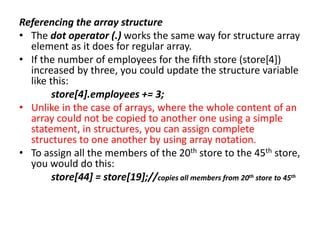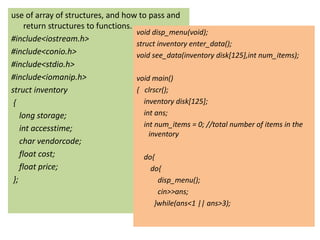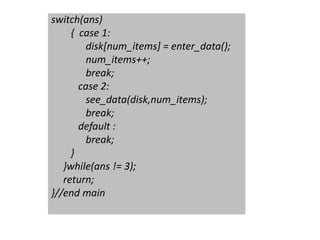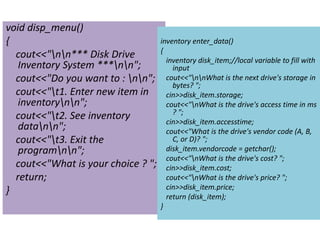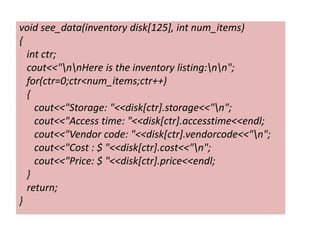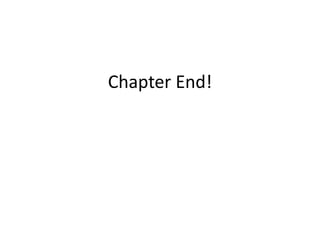1 de 56
Anúncio

### Chapter-Five.pptx

1. Chapter Five Arrays and Structures
2. What is Array • A collection of identical data objects, which are stored in consecutive memory locations under a common heading or a variable name. • An array is a group or a table of values referred to by the same name. • The individual values in array are called elements. • Array elements are also variables. • Set of values of the same type, which have a single name followed by an index. • In C++, square brackets appear around the index right after the name • A block of memory representing a collection of many simple data variables stored in a separate array element, and the computer stores all the elements of an array consecutively in memory.
3. Properties of arrays: • Arrays are zero-bounded;  index of the first element is 0 and the last element is N-1, where N is the size of the array. Ex array, array,…array[N-1] • It is illegal to refer to an element outside of the array bounds. • Array can only hold values of one type Array declaration • Declaring the name, type and setting the number of elements in an array is called dimensioning the array. • The array must be declared before one uses. • In the array declaration one must define: – type of the array (i.e. integer, floating point, char etc.) – Name of the array (identifier) – number of elements in the array (total number of memory locations to be allocated or the maximum value of each subscript)
4. General syntax: dataTypename arrayname [array_size]; • expression array size must be a constant such as 10 or a symbolic constant declared before the array declaration, or • a constant expression such as 10*sizeof (int), for which the values are known at the time compilation takes place. • Note: array size cannot be a variable whose value is set while the program is running. • Ex. to declare an integer with size of 10 having a name of num is: int num ;
5. Initializing Arrays • Declaring an array of local scope (within a function) will not initialize, so its content is undetermined. • Declaring a global array (outside any function)  initialize all elements to zero. • Ex. Global array declaration: int day ; • every element of day will be set initially to 0:
6. • But additionally, when we declare an Array, we have the possibility to assign initial values to each one of its elements using curly brackets { } . • For example: int day  = { 16, 2, 77, 40, 12071 }; • Number of elements within { } must be equal or less than the length of elements within [ ]. • Note: If we have less number of items for the initialization, the rest will be filled with zero.
7. • C++ allows the possibility of leaving empty the brackets [ ], where the number of items in the initialization bracket will be counted to set the size of the array. int day [ ] = { 1, 2, 7, 4, 12,9 }; //size of day set to 6 • You can use the initialization form only when defining the array. You cannot use it later, and cannot assign one array to another once. I.e. int arr [ ] = {16, 2, 77, 40, 12071}; int ar ; ar[ ]={1,2,3,4};//not allowed arr=ar;//not allowed • Note: when initializing an array, we can provide fewer values than the array elements. E.g. int a  = {10, 2, 3}; in this case the compiler sets the remaining elements to zero.
8. Accessing and processing array elements • Any elements of array can be access individually for reading or modifying (like variable). • But, to access individual elements, index or subscript must be used. • The format is the following: name [ index ] ; • In c++ the first element has an index of 0 and the last element has an index, which is one less the size of the array (i.e. arraysize-1). • Thus, day is the first element and day is the last element. • Following the previous examples where day had 5 elements, we can use the following to refer to each element:
9. • Ex. To store the value 75 in the third element of the array variable day??? day = 75; //as the third element is found at index 2 • Ex. To pass value of the third element of the array day to the variable a: a = day; // is like any variable of type int • Thus, an array enables us to create a lot of variables of the same type with a single declaration . • If we write day , we would be accecing to the sixth element of day and therefore exceeding the size of the array. This might give you either error or unexpected value. • In C++ it is perfectly valid to exceed the valid range of indices for an Array, which can cause certain detectable problems, since they do not cause compilation errors but they can cause unexpected results or serious errors during execution.
10. The uses of square brackets [ ] in arrays: – One is to set the size of arrays during declaration – The other is to specify indices for a specific array element when accessing the elements of the array • We must take care of not confusing these two possible uses of brackets [ ] with arrays: Eg: int day;//declaration of a new Array day = 75; // access to an element of the Array • Other valid operations with arrays in accessing and assigning: int a=1; day=a; day[a]=5; int b= day [a+2]; day [day [a] ] = day  + 5;
11. • Eg: Arrays example ,display the sum of the numbers in the array #include <iostream.h> int day [ ] = {16, 2, 77, 40, 12071}; int n, result=0; void main () { for ( n=0 ; n<5 ; n++ ) { result += day[n]; } cout << result; }
12. Arrays as parameters • At some moment we may need to pass an array to a function as a parameter. • In order to admit arrays as parameters the only thing that we must do when declaring the function is to specify in the argument the base type for the array that it contains, an identifier and a pair of void brackets [] . For example, the following function: void procedure (int arg[ ]) • admits a parameter of type "Array of int " called arg . In order to pass to this function an array declared as: int myarray ; • it would be enough with a call like this: procedure (myarray);
13. Here you have a complete example: // arrays as parameters #include <iostream.h> #include <conio.h> void printarray (int arg[], int length) { for (int n=0; n<length; n++) cout << arg[n] << " "; cout << "n"; } void mult(int arg[], int length) { for (int n=0; n<length; n++) arg[n]=2*arg[n]; } void main () { int firstarray[] = {5, 10, 15}; int secondarray[] = {2, 4, 6, 8, 10}; printarray (firstarray,3); printarray (secondarray,5); mult(firstarray,3); cout<<”first array after being doubled isn”; printarray (firstarray,3); getch() }
14. • As you can see, the first argument (int arg[] ) admits any array of type int , whatever its length is, for that reason we have included a second parameter that informs the function the length of each array that we pass to it as the first parameter so that the for loop that prints out the array can have the information about the size we are interested about. • The function mult doubles the value of each element and the firstarray is passed to it. • After that the display function is called. • The output is modified showing that arrays are passed by reference. • To pass an array by value, pass each element to the function
15. String of Characters: What is String? • What type of variables used so far???… numerical variables?? int, float,… char • What if you need to represent successive characters, like words, sentences, names, texts, etc. • Until now we have only used them as constants, but not variables able to contain them. • In C++ there is no specific elementary variable type to store string of characters. • In order to fulfill this feature we can use arrays of type char, which are successions of char elements. • char is the one used to store a single character, for that reason arrays of them are generally used to make strings of single characters. • For example, the following array (or string of characters) can store a string up to 20 characters long. char name ;
16. • The maximum size of 20 characters is not required to be always fully used. • For example, name could store at some moment in a program either the string of characters "Hello" or the string "studying C++”. • Therefore, since the array of characters can store shorter strings than its total length, there has been reached a convention to end the valid content of a string with a null character, whose constant can be written as '0’.
17. H e l l 0 0 S t u d y i n g C + + 0 •We could represent name (an array of 20 elements of type char) storing the strings of characters "Hello" and "Studying C++" in the following way: •Notice how after the valid content it is included a null character ('0') in order to indicate the end of string. The empty cells (elements) represent indeterminate values.
18. Initialization of Strings • Because strings of characters are ordinary arrays they fulfill same rules as any array. • For example, if we want to initialize with predetermined values: char mystring[ ] = { 'H', 'e', 'l', 'l', 'o', '0' }; • string of characters have an additional way to initialize its values: using constant strings. – specified enclosed between double quotes ( “ “ ), for example: Eg: "the result is: " • is a constant string that we have probably used in some occasion. • Unlike single quotes ( ' ) which allow to specify single character constants, double quotes ( " ) are constants that specify a succession of characters. • These strings enclosed between double quotes have always a null character ( '0' ) automatically appended at the end.
19. • Therefore we could initialize the string mystring with values by any of these two ways: char mystring [ ] = { 'H', 'e', 'l', 'l', 'o', '0' }; char mystring [ ] = "Hello"; • In both cases the Array or string of characters mystring is declared with a size of 6 characters (elements of type char ): the 5 characters that compose Hello plus a final null character ( '0' ) which specifies the end of the string. • Note that the assignation of multiple constants like double- quoted constants ( " ) to arrays are only valid when initializing the array, that is, at the moment when declared. • The following expressions are not valid for arrays mystring="Hello"; mystring[] = "Hello"; mystring = { 'H', 'e', 'l', 'l', 'o', '0' };
20. • So remember: We can "assign" a multiple constant to an Array only at the moment of initializing it. • An array is simply a constant pointer pointing to an allocated block of memory. And because of this constant feature, the array itself cannot be assigned any value, but we can assign values to each of the elements of the array. • At the moment of initializing an Array it is a special case, since it is not an assignation, although the same equal sign ( = ) is used.
21. Assigning Values to Strings • Array of character can store values using assignment operators. But the following is not allowed. mystring=”Hello”; • This is allowed only during initialization. So, what would be valid is to assign a string of characters to an array of char using a method like this: mystring = 'H'; mystring = 'e'; mystring = 'l'; mystring = 'l'; mystring = 'o'; mystring = '0'; • But, this does not seem to be a very practical method.
22. • Generally for assigning values to an array (specifically to a string of characters), a series of functions like strcpy are used. • strcpy ( str ing c o py ) is defined in the ( string.h ) library and can be called the following way: strcpy ( string1 , string2 ); • Thus, copy the content of string2 into string1s • string2 can be either an array, a pointer, or a constant string , • So, a valid way to assign the constant string "Hello" to mystring : strcpy (mystring, "Hello");
23. For example: #include <iostream.h> #include <string.h> int main () { char MyName ; strcpy (MyName,"Abebe"); cout << MyName; return 0; }
24. // setting value to string #include <iostream.h> #include<conio.h> void namecopy(char dest[], char source[]) { int c = 0; while(source[c] != ‘0’) { dest[c] = source[c]; c++; } dest[c] = ‘0’; cout<< “n your name after copying : ”<<dest; } void main() { clrscr(); char name,dest; cout<< “n enter your name cin>>name; namecopy(dest,name); getch(); }
25. • Another frequently used method to assign values to an array is by using directly the input stream ( cin ). • When cin is used with strings of characters it is usually used with its getline method: cin.getline ( char buffer [], int length , char delimiter = ' n'); • Where – buffer is the address where to store the input (like an array) – length is the maximum length of the buffer (the size of the array) and – delimiter is the character used to determine the end of the user input, (the default newline or 'n' ).
26. • Example on how to use cin.getline with strings: // cin with strings #include <iostream.h> #include<conio.h> int main () { char mybuffer ; cout << "What's your name? "; cin.getline (mybuffer,100); cout << "Hello " << mybuffer << ".n"; cout << "Which is your favourite team? "; cin.getline (mybuffer,100); cout << "I like " << mybuffer << " too.n"; getch(); return 0; }
27. • If you remember the section about communication through console, you will remember that we used the extraction operator ( >> ) to receive data directly from the standard input. • This method can also be used instead of cin.getline with strings of characters. • For example, in our program, when we requested an input from the user we could have written: cin >> mybuffer;
28. Limitation of cin>>buffer; • receive single words (not complete sentences) – as delimiter any occurrence of a blank character, including spaces, tabulators, newlines and carriage returns. • It is not allowed to specify a size for the buffer. What makes your program unstable in case that the user input is longer than the array that will host it. • For these reasons it is recommendable that whenever you require strings of characters coming from cin you use cin.getline instead of cin >>
29. Converting strings to other types • Due to that a string may contain representations of other data types like numbers it might be useful to translate that content to a variable of a numeric type. • For example, a string may contain "1977" , but this is a sequence of 5 chars not so easily convertible to a single integer data type. • The cstdlib ( stdlib.h ) library provides three useful functions for this purpose: atoi: converts string to int type. atol: converts string to long type. atof: converts string to float type. • All of these functions admit one parameter and return a value of the requested type ( int , long or float ). • These functions combined with getline method of cin are a more reliable way to get the user input when requesting a number than the classic cin>> method:
30. // cin and ato* functions #include <iostream.h> #include <stdlib.h> #include<conio.h> int main() { clrscr(); char mybuffer; float price; int quantity; cout << "Enter price: "; cin.getline (mybuffer,100); price = atof (mybuffer); cout << "Enter quantity: "; cin.getline (mybuffer,100); quantity = atoi (mybuffer); cout<<"nafter conversion :n"; cout<<"nprice is : "<<price; cout<<"nquantity is : "<<quantity; cout << "nTotal price: " << price*quantity; getch(); return 0; }
31. Header Files ALLOC.H ASSERT.H BCD.H BIOS.H COMPLEX.H CONIO.H CTYPE.H DIR.H DIRENT.H DOS.H ERRNO.H FCNTL.H FLOAT.H FSTREAM.H GENERIC.H GRAPHICS.H IO.H IOMANIP.H IOSTREAM.H LIMITS.H LOCALE.H MALLOC.H MATH.H MEM.H PROCESS.H SETJMP.H SHARE.H SIGNAL.H STDARG.H STDDEF.H STDIO.H STDIOSTR.H STDLIB.H STREAM.H STRING.H STRSTREA.H SYSSTAT.H SYSTIMEB.H SYSTYPES.H TIME.H VALUES.H
32. Functions to manipulate strings • The cstring library ( string.h ) defines many functions to perform some manipulation on strings. a. String length • Returns the length of a string, not including the null character (0). strlen (const char* string ); b. String Concatenation: • Appends src string at the end of dest string. Returns dest. • Has two forms, – Appending the whole content of the source strcat (char* dest , const char* src ); – Appending part of the source strncat (char* dest , const char* src, int size ); • Where size is the number of characters to be appended
33. c. String Copy: • Overwrites the content of the dest string by the src string. Returns dest. • Has two forms, – Copy the whole content of the source strcpy (char* dest , const char* src ); – Appending part of the source strncpy (char* dest , const char* src, int size ); • Where size is the number characters to be copied
34. d. String Compare: • Compares the two string string1 and string2. • Has two forms, – Compare the whole content of two strings strcmp (const char* string1 , const char* string2 ); – Compare only part of two strings strncmp (const char* string1 , const char* string2, int size ); • Where size is the number characters to be compaired • The compare function returns three different values: – returns 0 (if the strings are equal) – returns negative int(if the first is less than the second string) – returns positive int (if the first is greater than the second string
35. Multidimensional Arrays • It is arrays of arrays. • Ex. a bi-dimensional array can be imagined as a bi-dimensional table of a uniform concrete data type type multid_array_name[row_size][col_size];
36. • Matrix represents a bi-dimensional array of 3 per 5 values of type int . • The way to declare this array would be: int matrix   ; • Ex. the way to reference the second element vertically and fourth horizontally would be: matrix;
37. • Multidimensional arrays are not limited to two indices (two dimen). • They can contain so many indices as needed, although it is rare to have to represent more than 3 dimensions. • How much amount of memory required??? char century ; • Assigns a char for each second contained in a century, that is more than 3 billion chars ! What would consume is about 3000 megabytes of RAM memory if we could declare it? • Multidimensional arrays are nothing else than an abstraction, since we can simply obtain the same results with a simple array by putting a factor between its indices: int matrix ; is equivalent to int matrix ; (3 * 5 = 15)
38. //one dimensional array #include <iostream.h> int matrix ; int n; int main () { for (n=0;n<15;n++) matrix [n]=(n+1); return 0; } //multidimensional array #include <iostream.h> #define WIDTH 5 #define HEIGHT 3 int matrix [HEIGHT][WIDTH]; int n,m; int main () { for (n=0;n<HEIGHT;n++) for (m=0;m<WIDTH;m++) { matrix [n][m]=(n+1)*(m+1); } return 0; }
39. • In case that we decided to enlarge the array to a height of 4 instead of 3 it would be: #define HEIGHT 3 by the following code #define HEIGHT 4 • The above program assign values to the memory block called matrix in the following way:
40. Structures
41. • A structure is a collection of one or more variable types grouped together. • Structure referred using a single name (group name) and a member name. • Structure can be refer as a single variable, and • You also can initialize, read and change the parts of a structure (the individual variables that make it up). • Each element (called a member) in a structure can be of different data type.
42. struct [structure tag] { Member definition; Member definition; … Member definition; }[one or more structure variables]; //Example 1: struct Student { char ID; char FName; char LName; char Sex; int age; float CGPA; }; //Example 2: Struct Inventory{ char description; char part_no; int quantity; float cost; }; // all structures end with semicolon
43. • The above “Student” structure is aimed to store student record with all the relevant details. • After the definition of the structure, one can declare a structure variable using the structure tag. • If we need two variables to have the above structure property then the declaration would be: struct Inventory inv1,inv2; //or struct Student Stud1,stud2,Stud3; • Structure tag is not a variable name. • A structure tag is simply a label for the structure’s format. • The structure tag Inventory informs C++ that the tag called Inventory looks like two character arrays followed by one integer and one float variables. • A structure tag is like programmer defined data type.
44. Referencing members of a structure • To refer to the members of a structure use dot operator (.) • The General syntax:: VarName.Member; • Where VarName is the varaibale name of the structure variable and Member is varaibale name of the members of the structure Eg:- For the above student structure: • struct Student Stud; //declaring Stud Student structure strcpy(Stud.FName,”Abebe”); //assigned Abebe as First Name Stud.CGPA=3.21; //assignes 3.21 as CGPA value of Abebe sout<<Stud.FName; //display the name sout<<Stud.CGPA; // display the CGPA of Abebe
45. Initializing Structure Data • You can initialize members when you declare a structure, or you can initialize a structure in the body of the program. • Here is a complete program. …. struct cd_collection { char title; char artist; int num_songs; float price; char date_purchased; } cd1 = {"Red Moon","Sams and Sneeds", 12,11.95,"08/13/93"};
46. cout<<"nhere is the info about cd1"<<endl; cout<<cd1.title<<endl; cout<<cd1.artist<<endl; cout<<cd1.num_songs<<endl; cout<<cd1.price<<endl; cout<<cd1.date_purchased<<endl;
47. • A better approach to initialize structures is to use the dot operator(.). • the dot operator(.) is one way to initialize individual members of a structure variable in the body of your program. • The syntax of the dot operator is : structureVariableName.memberName;
48. here is an example: #include<iostream.h> . . . . void main() { clrscr(); struct cd_collection{ char title; char artist; int num_songs; float price; char date_purchased; }cd1; //initialize members here strcpy(cd1.title,"Red Moon Men"); strcpy(cd1.artist,"Sams"); cd1.num_songs= 12; cd1.price = 11.95; strcpy(cd1.date_purchased,"22/12/02"); //print the data cout<<"nHere is the info"<<endl; cout<<"Title : "<<cd1.title<<endl; cout<<"Artist : "<<cd1.artist<<endl; cout<<"Songs : "<<cd1.num_songs<<endl; cout<<"Price : "<<cd1.price<<endl; cout<<"Date purchased : "<<cd1.date_purchased; getch(); }
49. Arrays of Structures • Arrays of structures are good for storing a complete employee file, inventory file, or any other set of data that fits in the structure format. • Consider the following structure declaration: struct Company { int employees; int registers; double sales; }store; • Declare 1,000 store structures with the definition of the Company structure, each one containing three members. • NB. Be take care of resource limitation.(imagine RAM 64MB)
50. Array of structures can be declared after the declaration of the structure. struct Company { int employees; int registers; double sales; }; /*no structure variables defined yet*/ #include<iostream.h> … void main() { struct Company store; /*the variable store is array of the structure Company*/ … }
51. Referencing the array structure • The dot operator (.) works the same way for structure array element as it does for regular array. • If the number of employees for the fifth store (store) increased by three, you could update the structure variable like this: store.employees += 3; • Unlike in the case of arrays, where the whole content of an array could not be copied to another one using a simple statement, in structures, you can assign complete structures to one another by using array notation. • To assign all the members of the 20th store to the 45th store, you would do this: store = store;//copies all members from 20th store to 45th
52. use of array of structures, and how to pass and return structures to functions. #include<iostream.h> #include<conio.h> #include<stdio.h> #include<iomanip.h> struct inventory { long storage; int accesstime; char vendorcode; float cost; float price; }; void disp_menu(void); struct inventory enter_data(); void see_data(inventory disk,int num_items); void main() { clrscr(); inventory disk; int ans; int num_items = 0; //total number of items in the inventory do{ do{ disp_menu(); cin>>ans; }while(ans<1 || ans>3);
53. switch(ans) { case 1: disk[num_items] = enter_data(); num_items++; break; case 2: see_data(disk,num_items); break; default : break; } }while(ans != 3); return; }//end main
54. void disp_menu() { cout<<"nn*** Disk Drive Inventory System ***nn"; cout<<"Do you want to : nn"; cout<<"t1. Enter new item in inventorynn"; cout<<"t2. See inventory datann"; cout<<"t3. Exit the programnn"; cout<<"What is your choice ? "; return; } inventory enter_data() { inventory disk_item;//local variable to fill with input cout<<"nnWhat is the next drive's storage in bytes? "; cin>>disk_item.storage; cout<<"nWhat is the drive's access time in ms ? "; cin>>disk_item.accesstime; cout<<"What is the drive's vendor code (A, B, C, or D)? "; disk_item.vendorcode = getchar(); cout<<"nWhat is the drive's cost? "; cin>>disk_item.cost; cout<<"nWhat is the drive's price? "; cin>>disk_item.price; return (disk_item); }
55. void see_data(inventory disk, int num_items) { int ctr; cout<<"nnHere is the inventory listing:nn"; for(ctr=0;ctr<num_items;ctr++) { cout<<"Storage: "<<disk[ctr].storage<<"n"; cout<<"Access time: "<<disk[ctr].accesstime<<endl; cout<<"Vendor code: "<<disk[ctr].vendorcode<<"n"; cout<<"Cost : \$ "<<disk[ctr].cost<<"n"; cout<<"Price: \$ "<<disk[ctr].price<<endl; } return; }
56. Chapter End!
Anúncio